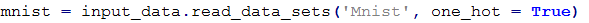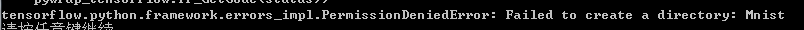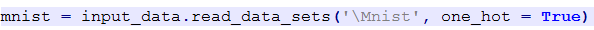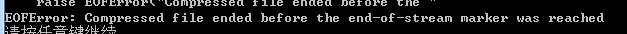# 自己的TensorFlowd的mnist入门

这是我关于mnist手势数据集的入门，包含了自己的一些感想，也是第一篇博客，希望能得到大家的指正，共同交流。

mnist是机器学习的入门水平，相当于编程的Holle world，但就是看似简单的东西，由于自己的水平有限，耗费了不少时间。

1. 自己一直用的是notepad++，但是在调用下载数据集时，会出现如下错误：2. 为此我打算自己下载数据集，下载地址  http://yann.lecun.com/exdb/mnist/  我将其存放在Mnist文件夹下，需要注意的一点是不要解压，运行时，出现错误，找了许多方法也不管用

没办法只能病急乱投医了，，，于是在命令行窗口将源代码跑了一遍，竟然运行成功了，，，，，吐血，，

首先导入tensorflow和其自带的input_data.py文件

import tensorflow as tf
from tensorflow.examples.tutorials.mnist import input_data

接下来运行下面一行代码下载数据集并获取（如果已经下载则直接获取数据集）

mnist = input_data.read_data_sets('Mnist', one_hot = True)

此时获取的数据集就保存在当前目录下的Mnist文件夹

然后运行如下代码构建tensorflow的计算图

#x没有指定具体样本数量，后面可以灵活插入具体的样本数量值
x = tf.placeholder("float", [None, 784])
W = tf.Variable(tf.zeros([784,10]))
b = tf.Variable(tf.zeros())
y = tf.nn.softmax(tf.matmul(x,W) + b)
y_ = tf.placeholder("float", [None,10])
cross_entropy = -tf.reduce_sum(y_*tf.log(y))
#优化算法采用梯度下降法
#初试化所有变量（tensorflow中必须初始化一下）
init = tf.initialize_all_variables()

下面创建Session来运行上面创建的计算图

sess = tf.Session()
sess.run(init)
#用for循环进行1000此梯度下降迭代
for i in range(1000):
#此处采用batch为100的随机梯度下降算法
batch_xs, batch_ys = mnist.train.next_batch(100)
sess.run(train_step, feed_dict={x: batch_xs, y_: batch_ys})
#用equal函数进行正确率的计算
correct_prediction = tf.equal(tf.argmax(y,1), tf.argmax(y_,1))
accuracy = tf.reduce_mean(tf.cast(correct_prediction, "float"))
#将正确率打印出来
print(sess.run(accuracy, feed_dict={x: mnist.test.images, y_: mnist.test.labels}))

我得到的结果是0.9058，其实这个结果对于mnist来说不是特别好，主要是因为只用了一层的NN，如果采用多层的CNN效果会好得多

posted @ 2018-12-07 10:45 Freddy520 阅读(...) 评论(...) 编辑 收藏